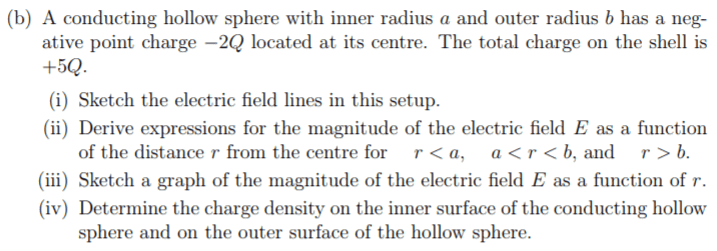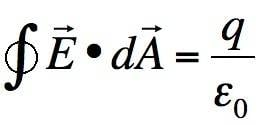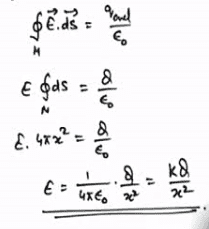# Conducting sphere Find the electric filed for r<a,a<r<b,r>b

Jozefina Gramatikova

## Homework Statement## Homework Equations## The Attempt at a Solution

for part ii)
a<r<b E=0
I am not sure what will be the difference between the formulas for the electric field for a<r and a>b I think the formulas will look the same:The only difference that I can think of is that when r<a, we are going to use the charge enclosed in that surface. For r>b we are going to use the total charge. Is that true?

#### Attachments

•